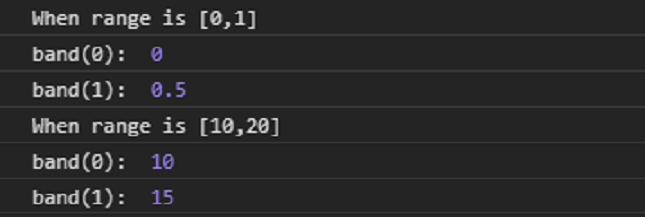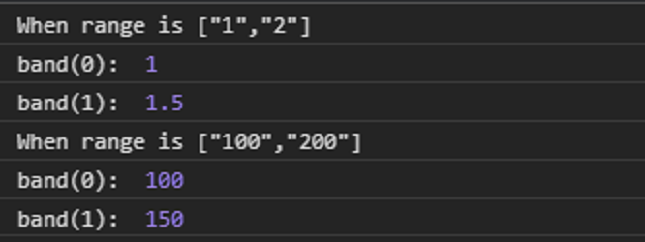# D3.js band.range() Function

• Last Updated : 23 Sep, 2020

The band.range() function in D3.js library is used to set the range of the scale to the specified two-element array of numbers. The default value of the range is [0, 1].

Syntax:

Hey geek! The constant emerging technologies in the world of web development always keeps the excitement for this subject through the roof. But before you tackle the big projects, we suggest you start by learning the basics. Kickstart your web development journey by learning JS concepts with our JavaScript Course. Now at it's lowest price ever!

```  band.range([range]);
```

Parameters: This function accepts single parameters as given above and described below.

• range: This parameter accepts a two-element array of numbers.

Note: If the element in the range are not numbers then they are coerced to number.

Return Value: This function does not return anything.

Below given are a few examples of the function given above.

Example 1:

## HTML

 ```<``html` `lang``=``"en"``>`` ` `<``head``>``    ``<``meta` `charset``=``"UTF-8"` `/>``    ``<``meta` `name``=``"viewport"` `path1tent``=``        ``"width=device-width, initial-scale = 1.0"` `/>`` ` `    ``<``script` `src``=``"https://d3js.org/d3.v4.min.js"``>``    `````` ` `<``body``>``    ``<``script``>``        ``// Create the band scale with specified``        ``// domain and range.``        ``var band = d3.scaleBand()``            ``.domain([0, 1])``            ``.range([0, 1]);`` ` `        ``console.log("When range is [0, 1]");``        ``console.log("band(0): ", band(0));``        ``console.log("band(1): ", band(1));`` ` `        ``var band = d3.scaleBand()``            ``.domain([0, 1])``            ``.range([10, 20]);`` ` `        ``console.log("When range is [10, 20]");``        ``console.log("band(0): ", band(0));``        ``console.log("band(1): ", band(1));``    `````` ` ``

Output:Example 2:

## HTML

 ```<``html` `lang``=``"en"``>`` ` `<``head``>``    ``<``meta` `charset``=``"UTF-8"` `/>``    ``<``meta` `name``=``"viewport"` `path1tent``=``        ``"width=device-width, initial-scale = 1.0"` `/>`` ` `    ``<``script` `src``=``"https://d3js.org/d3.v4.min.js"``>``    `````` ` `<``body``>``    ``<``script``>``        ``// Create the band scale with specified ``        ``// domain and range.``        ``var band = d3.scaleBand()``            ``.domain([0, 1])``            ``// These chars are coerced to numbers``            ``.range(["1", "2"]);`` ` `        ``console.log("When range is [\"1\", \"2\"]");``        ``console.log("band(0): ", band(0));``        ``console.log("band(1): ", band(1));`` ` `        ``var band = d3.scaleBand()``            ``.domain([0, 1])``            ``// These chars are coerced to numbers``            ``.range(["100", "200"]);`` ` `        ``console.log("When range is [\"100\", \"200\"]");``        ``console.log("band(0): ", band(0));``        ``console.log("band(1): ", band(1));``    `````` ` ``

Output:My Personal Notes arrow_drop_up